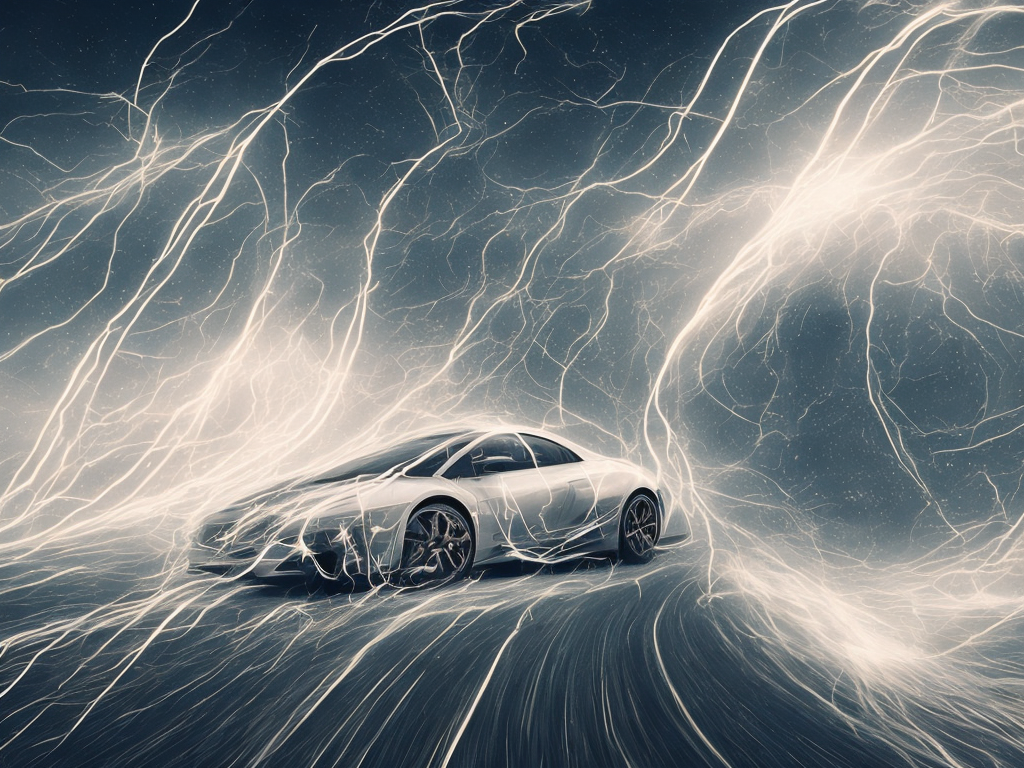# Difference Between Velocity And Speed

tl;dr
Velocity is a vector quantity that takes direction into account, while speed is a scalar quantity that only describes the magnitude of the displacement of an object over time.Velocity and speed are two terms that are often used interchangeably when it comes to describing how fast an object is moving. However, there is a significant difference between the two terms, and understanding this difference is essential to understand the basic concepts of physics.

Speed can simply be defined as the rate at which an object moves. It is a scalar quantity that describes the magnitude of the displacement of an object over time. The formula to calculate speed is given by:

Speed = Distance/Time

Thus, if an object covers a distance of 100 meters in 10 seconds, then its average speed would be 10 meters per second. Speed can be expressed in kilometers per hour, miles per hour, or any other unit of distance per unit of time.

Velocity, on the other hand, is a vector quantity that describes the speed of an object in a particular direction. When an object moves in a straight line, its velocity is the same as its speed. However, if an object moves in a curved path or changes direction, then its velocity would change even if its speed remains the same.

Velocity is given by the formula:

Velocity = Displacement/Time

where displacement is defined as the change in position of an object, taking direction into account. The direction of velocity can be described using terms like North, South, East, West, Up, Down and by using positive or negative signs.

For example, suppose a car travels from point A to point B, a distance of 100 meters, in 10 seconds. Then the displacement of the car from point A to point B is the distance between the two points, which is 100 meters. If the car traveled directly from A to B, then the displacement would be equal to the distance traveled. In this case, the velocity of the car would be:

Velocity = Displacement/Time = 100/10 = 10m/s

However, suppose the car did not travel in a straight line and made a U-turn at some point. In that case, the distance traveled by the car would still be 100 meters, but the displacement would be less than 100 meters since it has to account for the change in direction. For example, suppose the car traveled 60 meters in one direction, then turned around and traveled another 40 meters in the opposite direction before reaching point B. Then the displacement of the car would be 20 meters, which is the distance between its final position and its starting position. In this case, its velocity would be:

Velocity = Displacement/Time = 20/10 = 2m/s

Thus, even though the speed of the car remained constant, its velocity changed due to the change in direction.

In general, speed is a scalar quantity, and velocity is a vector quantity. Scalar quantities are those that have only magnitude, while vector quantities have both magnitude and direction. Other examples of scalar quantities include distance, time, temperature, mass, and energy. On the other hand, vector quantities include displacement, velocity, acceleration, and force.

The significant difference between velocity and speed lies in the fact that velocity takes direction into account, while speed does not. For example, suppose two cars travel at the same speed, but one car is moving north while the other car is moving west. Then, their velocities would be different since they are moving in different directions.

The concept of velocity is used in many areas of physics, from classical mechanics to relativity. In classical mechanics, velocity is an essential parameter used to describe the motion of objects. In Newtonian mechanics, for example, the second law of motion states that the force applied to an object is directly proportional to its acceleration, which in turn is related to its velocity.

In relativity, the concept of velocity is used to describe the behavior of moving objects under different frames of reference. The theory of relativity predicts that the velocity of light is constant in all frames of reference, and that the faster an object moves, the more its mass increases and the more time is distorted.

In conclusion, velocity and speed are two concepts that are often used interchangeably, but they have different meanings in physics. Speed is a scalar quantity that describes how fast an object is moving, while velocity is a vector quantity that takes direction into account. Understanding the difference between velocity and speed is essential to grasp the basic concepts of physics, as well as to solve practical problems in engineering, science, and technology.